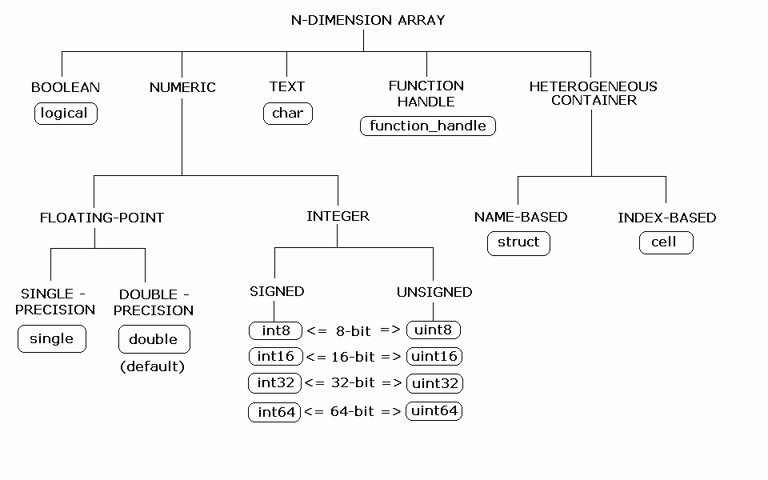## Data Conversion with C# and MATLAB Types

When the .NET client invokes a MATLAB® function through a server request and receives a result in the response, data conversion takes place between MATLAB data types and C# data types.

### Working with MATLAB Data Types

There are several data types, or classes, that you can work with in MATLAB. Each of these classes is in the form of a matrix or array. You can build matrices and arrays of floating-point and integer data, characters and strings, and logical true and false states. Structures and cell arrays provide a way to store dissimilar types of data in the same array.

Note

Function Handles are not supported by MATLAB Production Server™.

The fundamental MATLAB classes are circled in the following diagram.Each MATLAB data type has a specific equivalent in C#. For a mapping of these one-to-one relationships, see Conversion Between MATLAB Types and C# Types.

### Scalar Numeric Type Coercion

Scalar numeric MATLAB types can be assigned to multiple .NET numeric types as long as there is no loss of data or precision.

The following table describes the type compatibility for scalar numeric coercion.

MATLAB to .NET Numeric Type Compatibility

MATLAB Type.NET TypesC# Type
`uint8``System.Byte`, `System.Int16`, `System.UInt16`, `System.Int32`, `System.UInt32`, `System.Int64`, `System.UInt64`, `System.Single`, `System.Double``byte`
`int8``System.SByte`, `System.Int16`, `System.Int32`, `System.Int64`, `System.Single`, `System.Double``sbyte`
`uint16``System.UInt16`, `System.Int32`, `System.UInt32`, `System.Int64`, `System.UInt64`, `System.Single`, `System.Double``ushort`
`int16``System.Int16`, `System.Int32`, `System.Int64`, `System.Single`, `System.Double``short`
`uint32``System.UInt32`, `System.Int64`, `System.UInt64`, `System.Single`, `System.Double``uint`
`int32``System.Int32`, `System.Int64`, `System.Single`, `System.Double``int`
`uint64``System.UInt64`, `System.Single`, `System.Double``ulong`
`int64``System.Int64`, `System.Single`, `System.Double``long`
`single``System.Single`, `System.Double``float`
`double``System.Double`, `System.SByte`, `System.Byte`, `System.Int16`, `System.UInt16`, `System.Int32`, `System.UInt32`, `System.Int64`, `System.UInt64`, `System.Single``double`

### Dimension Coercion

In MATLAB, dimensionality is an attribute of the fundamental data types and does not add to the number of data types as it does in .NET. For example, in C#, `double`, `double[]` and `double[,]` are three different data types. In MATLAB, there is only a `double` data type and possibly a scalar instance, a vector instance, or a multi-dimensional instance. For example, if your MATLAB function returns a three-dimensional double array, your C# function declares its return value as a C# three-dimensional `double` array

C# SignatureValue Returned from MATLAB
`double[,,] foo() ``ones(1,2,3)`

How you define your MATLAB function and the corresponding C# method signature determines if your output data will be coerced, using padding or truncation.

The .NET client API performs the coercion automatically for you. This section describes the rules followed for padding and truncation.

Note

Multidimensional arrays of C# types are supported. Jagged arrays are not supported.

When the return type of a C# method has a greater number of dimensions than that of a MATLAB method, the dimensions of the MATLAB return type are padded with ones (`1`s) to match the required number of output dimensions in C#.

The following table provides examples of the padding. For example, when your MATLAB function returns a two-dimensional matrix but your C# function declares a four-dimensional array return value, the MATLAB matrix becomes a four-dimensional array with two trailing singular dimensions.

MATLAB FunctionC# Method SignatureDimensions in MATLABDimensions in C#

`function a = foo`

``` a = ones(2,3);```

`double[,,,] foo() ``size(a)` is `[2,3]`Array will be returned as size `2,3,1,1`

#### Truncation

When return type of a C# method has fewer dimensions than the dimensions of the return type of a MATLAB method, the dimensions of the MATLAB return type are truncated to match the required number of output dimensions in C#. This is only possible when extra dimensions for MATLAB array have values of ones (`1`s) only.

To compute appropriate number of dimensions in C#, excess ones are truncated in the following order:

1. From the end of the array

2. From the beginning of the array

3. From the middle of the array (scanning front-to-back).

The following tables provide examples of truncation.

How MATLAB Truncates Your C# Method Return Type

MATLAB FunctionC# Method Signature Dimensions in MATLABDimensions in C#

`function a = foo`

``` a = ones(1,2,1,1,3,1);```

`double[,] foo()``size(a)` is `[1,2,1,1,3,1]`Array will be returned as size `2,3`

Following are some examples of dimension shortening using the `double` numeric type.

Truncating Dimensions in MATLAB and C# Data Conversion

MATLAB Array DimensionsDeclared Output C# TypeOutput C# Dimensions
`1 x 1``double`0 (scalar)
`2 x 1``double[]``2`
`1 x 2``double[]``2`
`2 x 3 x 1``double[,]``2 x 3`
`1 x 3 x 4``double[,]``3 x 4`
`1 x 3 x 4 x 1 x 1``double[,,]``1 x 3 x 4`
`1 x 3 x 1 x 1 x 2 x 1 x 4 x 1``double[,,,]``3 x 2 x 1 x 4`

### Empty (Zero) Dimensions

#### Passing C# Empties to MATLAB

When a `null` is passed from C# to MATLAB, it will always be marshaled into `[]` in MATLAB as a zero by zero (`0 x 0`) `double`. This is independent of the declared input type used in C#. For example, all the following methods can accept `null` as an input value:

```void foo(String input); void foo(double[] input); void foo(double[,] input); ```

And in MATLAB, `null` will be received as:

```[] i.e. 0x0 double ```

#### Passing MATLAB Empties to C#

An empty array in MATLAB has at least one zero (`0`) assigned in at least one dimension.

For example, you can return an empty array from `function a = foo` by assigning `a` to any of the following values:

``` a = []; a = ones(0); a = ones(0,0); a = ones(1,2,0,3); ```

Empty MATLAB data is returned to C# as `null` for all the above cases.

For example, in C#, the following signatures return `null` when a MATLAB function returns an empty array.

```double[] foo(); double[,] foo(); ```